## General Question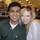# Factoring out the square root?

Asked by Sloane2024 (1879) August 28th, 2008

I’m incredibly and profusely sorry for the “homework” question, but I’ve searched all my available sources for an answer to my inquiry. I have a trig test tomorrow and am utterly confused on how to factor out a square root. The exact problem is finding if there is any number that will factor out of 448. This isn’t the entire problem, considering that I must find the 6 trigonometric functions of a 30–60-90 triangle, so this isn’t a scenario of helping me “slide off easy”. I honestly need the help, and any demonstrations or links would be immensely appreciated. Thank you.

Observing members: 0Composing members: 0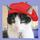I am doing this on my fingers; the square roots that factor out seem to be 1, 4, and 64. Can’t you simply memorize the trig functions of a 30–60-90 triangle?

gailcalled (54644)“Great Answer” (1) Flag as…I know the trig functions and ratios of both the 30–60-90 and the 45–45-90, but knowing how to factor out square roots in general will be vital for the success of this test. So, I guess what I’m really asking for is a mini lesson in factoring, lol.

Sloane2024 (1879)“Great Answer” (0) Flag as…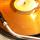Factoring is just figuring out what number a number is divisible by. So 448 is even so it is divisible by 2.
448 = 2 * 224. 224 is also even so…
448 = 2 * 2 * 112. 112 is even so….
448 = 2 * 2* 2 * 56. 56 is even so…
448 = 2 * 2* 2 * 2* 28. 28 is even so…
448 = 2 * 2* 2 * 2* 2* 14. 14 is even so…
448 = 2 * 2* 2 * 2* 2* 2* 7. seven is prime. so we are done factoring

Do you need more help?

mvgolden (824)“Great Answer” (2) Flag as…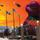Wolfram Research has some of the most detailed math concept explanations known to man! This is a link to everything they have on trigonometric functions.

lookup -
sin,cos,tan,
csc,sec,cot

Do some research on what these functions are and you should have no problems! Keep in mind that these explanations give the calculus derivations, so have fun!

@mvgolden, your factoring lesson was superb; except for one small thing:
instead of regarding each “even” number as factorable, you should regard each as non-prime. For example, if 27 is a remaining number: 9*3 = 27; the 9 can be rooted out. The key word here is non-prime.

You must also remember to take out the factors that can be rooted out. In this case, take similar groups of 2.
Consider the last step provided by @mvgolden:
`448=2*2*2*2*2*2*7`
you are taking the ^2 root. In this case you take out “groups of 2.”
in the case of cube root (^3), take out groups of 3
So you really have:
`sqrt(448) = sqrt(2*2 * 2*2 * 2*2 * 7)`
`sqrt(448) = sqrt( 4 * 4 * 4 * 7)`
`sqrt(448) = 2*2*2*sqrt(7)`
`sqrt(448) = 8sqrt(7)`

Does this make sense to you?

blastfamy (2174)“Great Answer” (2) Flag as…That really sounds like fun.

gailcalled (54644)“Great Answer” (1) Flag as…@My, wasn’t he asking for the square root factors, only? Not that I can remember much of this stuff.

gailcalled (54644)“Great Answer” (1) Flag as…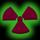If a number is a multiple, you can find all factors of the root, and find the biggest number that is a perfect square, and find what that multiplied by x and if x isn’t a perfect square, then it remains under the radical, and you evaluate the perfect square, and it’s still multiplied by the number under the radical.

ex: sqrt(48) = sqrt(16) * sqrt(3), so your final answer would be 4 * sqrt(3).

winblowzxp (498)“Great Answer” (1) Flag as…To clean it up:

√48 = √16 * √3 = 4√3

winblowzxp (498)“Great Answer” (1) Flag as…Wasn’t he asking for square root factors of 448? Or can you leave the number under the radical under the radical, if you get my drift?

gailcalled (54644)“Great Answer” (1) Flag as…Thank you SOOOOOOOOOOOOO much!! I can’t even begin to express my appreciation for all of your help! Lurve for everyone!! :D

Sloane2024 (1879)“Great Answer” (0) Flag as…@Sloane; you may understand, but I don’t. All those squirts make no sense for 448.

gailcalled (54644)“Great Answer” (0) Flag as…√448 = √16 * √28 = 4√28

If he doesn’t simplify square roots, then the teacher could take off points for it.

winblowzxp (498)“Great Answer” (0) Flag as…I’m a she, by the way, lol

Sloane2024 (1879)“Great Answer” (0) Flag as…@winblowzxp, funny how you stress the importance of simplifying the square root, yet you left…
`4sqrt(28)` unsimplified
`4sqrt(7*4)`
`2*4sqrt(7)`
`8sqrt(7)` should be the answer.

blastfamy (2174)“Great Answer” (0) Flag as…Eh, for some reason I thought it was prime. Hell, I’m surprised that I remembered how do do it.

winblowzxp (498)“Great Answer” (0) Flag as…Don’t worry. It’s like riding a bike…
Or eating ice cream…

It just comes through when you least expect it!

blastfamy (2174)“Great Answer” (0) Flag as…That much is true. Remembering how to do it without calculus is a bit tricky.

winblowzxp (498)“Great Answer” (0) Flag as…@Blast; I used only my fingers (see first answer) and came up with 64. I left out the (x 7) but that seemed obvious.

gailcalled (54644)“Great Answer” (0) Flag as…@Gail, yes. You are right. This kind of factoring is easy. It can, and should be done by exactly the method you described.

My thought is that the initial teaching to someone who does not understand the process entirely should be to explain with the most basic numbers (ruling out all error through possible mistakes over quickly coming to the wrong conclusions). Teaching the full-out factoring way lays the groundwork to solve such problems by the faster way that most people use.

The true utility in this method, however, comes when you are simplifying greater roots than the square. Were I trying to factor out something larger, say `1280^(1/7)`, it would be helpful to understand the fundamental concept of ‘any number that factors with a multiplicity of 7 can be factored out.’

I just think that the first way is an effective teaching tool that conveys the fundamentals of simplifying radicals more effectively.

blastfamy (2174)“Great Answer” (0) Flag as…@blast; you are right, I am sure. I don’t have enough fingers to check your math. (I grew up in the slide rule era. I still have the beautiful ivory one my father gave me.) Thank heavens for the scientific calculator. The days when I was a math student are long ago and far away.

gailcalled (54644)“Great Answer” (0) Flag as…I imagine that you’re taking the 7 root of 1280?

If I remember right, you can break up ‘n’ roots like exponents.

If you really want to get clever, you could do it logarithmically.

winblowzxp (498)“Great Answer” (0) Flag as…Do I have to? Please, can I not have some more? And I still have an old book of logs. The cat is probably sitting on it right now.

At the time, when I was at school, I found it all intellectually interesting but now it is a vague and distant memory. I seem to remember that log 100 in base 10 is 2. I then have to resort to fingers again. Another amazing mathematical discovery however.

You sound very comfortable with all this. Student, physicist, engineer, mathophile?

gailcalled (54644)“Great Answer” (0) Flag as…Engineer/mathophile

winblowzxp (498)“Great Answer” (0) Flag as…or